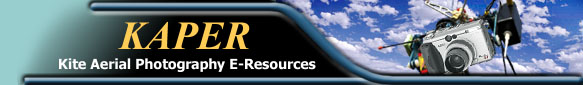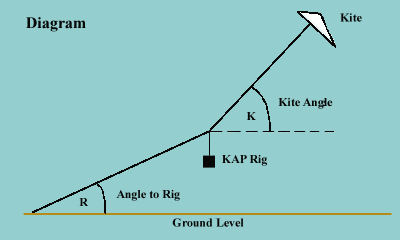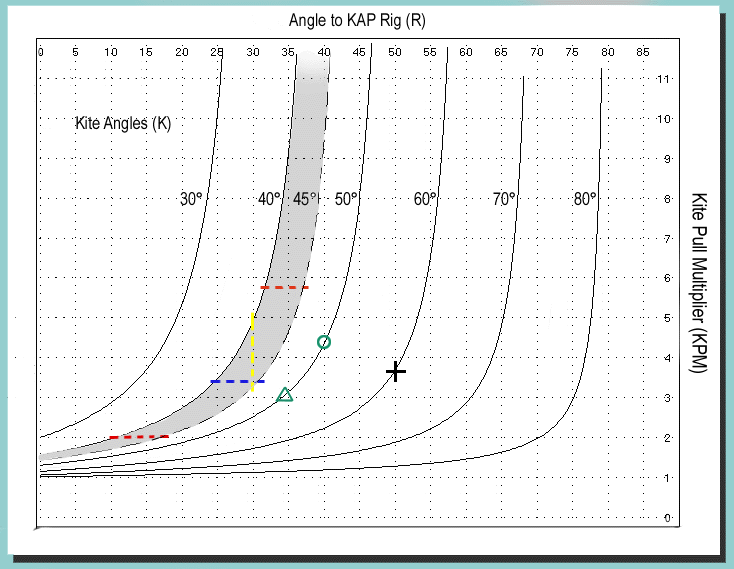# How Much Weight Can My Kite Lift?

[Ed- This page was modified March 2002 to make the concepts presented easier to understand and apply. Some of the implications from the graph should also be easier to understand.] Trying to figure out what type or size of kite to use for KAP has always been a formidable undertaking for the beginner. The charts on this page along with the information that is provided about each kite on the kite comparison chart page, sprinkled with some of the information on the Basics/Wind Speed page, should allow any KAPer to make a very educated decision about purchasing or building a particular kite, or about how heavy you can make your camera cradle.

## The DiagramThis diagram shows a typical KAP flying situation as it relates to the measurements on the graph below. To properly use the graph you need to refer to this diagram.

## The Graph

The graph below has 3 different parameters:
1. Kite Angle (angle K in diagram)
• Each type of kite has a different characteristic angle that it flies above the horizon. You can use the values listed on the Basic /Kites page, or establish your own values by flying your kite without any weight attached.
• Notice that kites that fly at higher angles require less pull to lift the same weight to the same angle. They will also exhibit more of the "Yo-Yo Effect"

2. Angle to Rig (angle R in diagram)
• The angle from the ground to the KAP Rig will always be less than the Kite angle. As the angle to the KAP Rig approaches the Kite angle the amount of pull necessary to lift the rig becomes very large.

You can demonstrate this principle by holding a length of kite line between your hands with a weight, close to that of your KAP cradle, hanging in the middle. Increasing the tension will cause the weight to rise. The amount of tension needed to completely remove the sag created by the weight becomes very hard if not impossible for most people. Completely removing the sag would be the same as angle R equaling angle K. In a kite setup the tension is supplied by the pull of the kite.

• It is interesting to note that, in the portions of the curves that are more horizontal, small changes in the kite pull represent large changes in the angle to the KAP Rig this is seen as the "Yo-Yo Effect" (see Basics / Kite Flying page)
The charts could be used to match the proper combination of KAP Rig weight and kite performance to minimize the "Yo-Yo Effect".

3. Kite Pull Multiplier
• Multiply the weight of your camera cradle by the kite pull multiplier to get the amount of pull need by the kite to lift the cradle to angle R.
• Some kite pull values are listed on the Basic /Kites page for a few kites.
• New kite pull values will be listed as they become available
EXAMPLE-On the graph below the curve marked with the "+" is for a kite that typically will fly at a 60° angle (angle K in diagram above). The horizontal graph scale indicates a 50° angle to the KAP Rig (angle R in diagram) and the vertical scale indicates that the kite pull multiplier is about 3.75. If you had a 1.25 pound camera cradle then multiply 3.75 times 1.25 to get 4.69 pounds of kite pull needed to lift your camera cradle to a 50° angle.
OR
Expressed mathematically;
For a given angle K and angle R,

## KPM × cradle weight = PullThe colored markings and the grayed area are part of the examples below.

The dashed curve representing 45° is an estimate and not calculated using the formula given below. It was added to the graph by hand and is used in one of the examples below.

## Different Ways To Use The Graph

1. Rank Beginner- If you don't have a kite or a camera cradle and you want to know where to start, first you need to know that there are no hard and fast answers, but there are guidelines that will get you started.

• Choose the type of cradle you will be flying a year from now. You might start with something lighter and more simple, but leave room to grow. Your choice will give you an idea of the amount of weight you will lift. If you do not have a camera cradle use these average values below.
• Manual Cradle, Disposable Camera ----- 1.00#  (454g)
• Remote Control, Point & Shoot Camera - 1.75# (794g)
• Remote Control, SLR Camera ----------- 2.50# (1134g)
• Chose a kite from the table on the Basics/kites/comparisons page. Using the graph above, calculate the limits of the kite's performance as they relate to the weight determined in the previous step.
STILL CONFUSED?
HERE ARE SOME OF EXAMPLES
Example, Approach One: You have a remote controlled P&S cradle weighing 1.75#(794g) and decided to evaluate the Flow Form 16 and 30 because you like the idea of a kite that requires no assembly in the field. The kite comparison table indicates that both Flow Forms fly at 40-45 degrees (the gray area of the chart). The kite comparison table has the amount of pull listed for 2Bft and 3Bft. The FF16 has a pull of 3.5# & 10# respectively and the FF30 has 6# & 23#.

We now know the weight of the cradle and the amount of pull of the kite. Using some very basic algebra we can now calculate the Kite Pull Multiplier or KPM (the value on the Y axis of the graph above).

KPM is calculated by dividing the kite pull by the weight of the cradle. The KPM for the FF16 is 2 & 5.7 respectively (Red Dashed Lines) and KPM for the FF30 3.4 & 13.1. (Blue Dashed Line is the lower KPM value for the FF30 the larger value is off the top of the graph).

The graph tells us that the FF16 would only be able to lift a 1.75# cradle to an angle between 10 and 17 degrees in 2Bft. winds, this is where the lower Red Line intersects the 40° and 45° curves. This would not be adequate for most KAP situations. The upper Red Line intersects the 40° and 45° curves at angles to the rig of 32° and 37° which is acceptable.

The decision you have to make is whether to buy the smaller FF and wait for stronger winds or buy the larger FF and be able to fly in 2Bft. winds.
[Ed-As a note, if I could not fly in 2Bft winds I would miss 70% of my KAP opportunities in this part of the country.]

Example, Approach Two: You want your kite to lift your camera cradle to an minimum angle of 30°. The total weight suspended from the line is 1.75#. You want to decide if a FF16 or a FF30 would be better for your situation. The Yellow Dashed Line on the chart above represents the minimum angle that you chose.

The KPM ranges from 3.4 to 5.0. Multiplying these values by 1.75# will give the range of kite pull need to lift the cradle to the chosen minimum angle of 30°, 5.95#(2699g) to 8.75#(3969g).

Looking at the kite comparison table the FF30 will be able to lift the cradle to a 30° angle in winds of 2Bft. (the low end of both Flow Form's operating range). The FF16 will only be able to meet the minimum if the winds are near 3Bft. You've reached the same conclusion as the example above but with a different approach.

2. Seasoned KAPer- You have flown your P&S R/C cradle a couple of dozen times and now you want to step up to an SLR cradle. Your favorite kite has a typical flying angle (K) of 60° and pulls about 4.5#(2041g) in 2Bft. winds. (Remember to always measure the pull of the kite without any weight attached to the line.)

You want to know how heavy your new cradle can be and still give acceptable results with your favorite kite. From your vast repository of practical KAP knowledge you set the minimum acceptable performance at an angle to the Cradle (R) of 35°.

The 60° curve intersects the 35° line at a KPM value of 2.9. The measured kite pull of 4.5# divided by 2.9 equals 1.55#(703g) which would be the maximum weight allowed to meet these minimum qualifications.

This information tells you that the only way that you would possibly be able to lift a SLR, to 35°, in 2Bft. winds, with this kite, is using a very light SLR camera and a manual cradle. A R/C cradle for a SLR camera will be over 2#(907g). You have to make a decision, use a manual cradle, get a bigger kite, or build a heavier R/C cradle and wait for stronger winds.

3. Finding Your Kite's Pull Without Scales- Like the Seasoned KAPer above you would like to know if your kite could lift a heavier rig. You are using a kite that does not have any data listed on the KAPER kite comparison table. You don't have a wind meter or an electronic fish scale to make accurate measurements of your kite's performance. (DO NOT try to save money and use spring-type fish scales. They are NOT accurate.) You will have to measure the angles R and K , so you invest 70¢ in a plastic protractor and attach a short plumb line to the center point. You find a location to fly your kite that has a flag pole very nearby. You have also studied the flag animations on the KAPER Wind Speed page (this will not work for wind speeds over 3 Bft.)

First you fly your kite without the rig attached and take several measurements of the kite's angle of flight (angle K on the diagram). You then attach your rig, or an equivalent weight, and let out some line. The rig doesn't have to be out very far, 15 feet(4.5m) would be sufficient. Watching the nearby flag you record the angle R along with the Beaufort number that you estimated by watching the flag. Do this several times until you are satisfied that you are getting consistent results. After several measurements you have the following information:
• Angle K = 50°
• Angle R = 40°
• Wind Speed = 2Bft.
This is represented by the Green Circle on the graph above. Reading the value on the y-axis you get a KPM of 4.4 (KPM is a pure number, there are no units associated with it.). Your rig weighs 1.75# so using the formula above the graph you write the equation 1.75 × 4.4 = 7.7# of pull.

You want to build a new rig that you estimate will be 2.5# and wish to know how it will perform with this kite. The KPM value of 4.4 is good for your lighter rig but you can not use that value for the heavier rig.

You do know that your kite flies at 50° and that it pulls 7.7# in a 2 Bft wind.
Pull ÷ Weight = KPM so 7.7# ÷ 2.5# = 3.08
and this is represented by the Green Triangle on the 50° curve. The angle R for this KPM is about 34°. This means that this kite will lift a 2.5# rig to an angle of 34° in a 2 Bft. wind, which is a workable combination.

## FORMULA

If you desire to calculate curves specifically for your own KAP equipment, the formula is given below. By substituting the actual weight of your camera cradle into the equation the kite pull will be the exact amount needed to lift your equipment. You can use pounds or grams, but don't mix units. Your answer will be in the units you use for the weight of your cradle.

Kite Pull = (sin K)WR + ((cos K )WR / tan (K - R))

K = Kite angle in degrees
WR = Weight of KAP Rig (you choose the units, Metric or English)
R = Angle to KAP Rig in degrees

This formula is derived from vector analysis of the diagram at the top of the page. If you attempt to measure your kite's pull, measure it without any weight attached to the line.

Remember that a particular kite does not always fly at the same angle K, the angle will vary from one moment to the next, but it will have an average or typical angle at which it flies.

## A NOTE ABOUT UNITS of WEIGHT

Since there are no weight units associated with the graph, this graph can be use to calculate kite pull in any unit of measure. The units you use to weigh your camera cradle will be the units of kite pull after you use the kite pull multiplier.